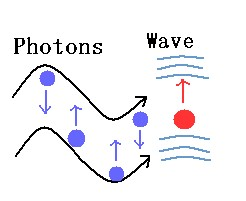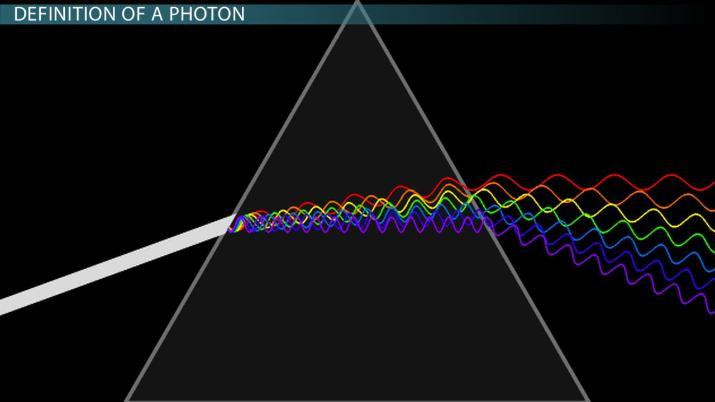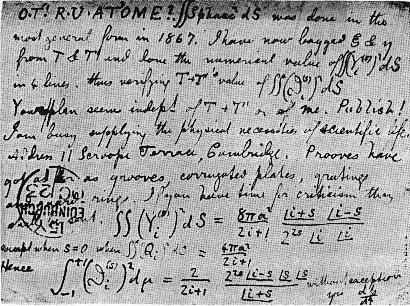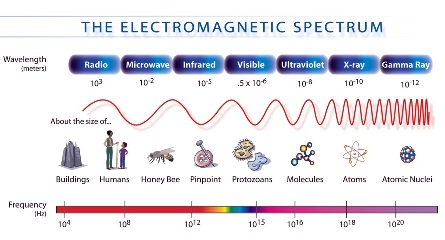# What is a Photon?

Nicholas Amendolare, Thomas Zesiger
• Author
Nicholas Amendolare

Nicholas Amendolare is a high school and middle school science teacher from Plymouth, Massachusetts. He has a bachelor's degree in environmental science from Worcester Polytechnic Institute and a master's degree in education from Harvard University. He has been a teacher for nine years, has written for TED-Ed, and is the founder of www.MrAscience.com.

• Instructor
Thomas Zesiger

Thomas has taught electronics and communications engineering, math, and physics and has a master's degree in electrical engineering.

What is a photon? Learn about photon, photon emission and the properties of photon wavelengths, and delve into the complexities of photon energy. Updated: 09/09/2021

Show

## What is a Photon?

A photon is a tiny particle made up of electromagnetic waves. They have no mass and no charge. You can think of them as a tiny packet of light energy.

A photon is an example of a quantum, a discrete packet of energy or matter. Strangely, photons have properties of both particles and waves. They have momentum and they behave as a concentrated unit, like particles. But they have a specific frequency and wavelength, like a wave. Einstein himself was mystified by this wave-particle duality, saying, "It seems as though we must use sometimes the one theory and sometimes the other... We have two contradictory pictures of reality; separately neither of them fully explains the phenomena of light, but together they do."

### Photon Definition

The scientific definition of a photon is a particle representing a quantum of light, or other electromagnetic radiation, that carries energy proportional to its frequency. Photons make up all of the light we can see. A rainbow, for example, is reflecting red, orange, yellow, green, and blue photons, all with their own distinct color. But there are many photons that the human eye cannot see as well. Infrared photons are sometimes used to help keep our food warm, and ultraviolet photons can give us sunburns. In all cases, the photon is a discrete quantum of light vibrating at a very specific frequency.

### Maxwell's Equations

Our modern understanding of light, including its behavior as both a particle and a wave, can be described by the equations of James Clerk Maxwell, published in 1864. The equations themselves are much too advanced to briefly discuss, but suffice it to say, they show that electric and magnetic fields obey wave equations. His equations also revealed that the speed of light was equal to 2.998x{eq}10^8 {/eq} m/s. Because the speed of light had already been measured experimentally, Maxwell's theories fit the current understanding of the behavior of light while also paving the way for the future. The detection and production of radio waves, for example, was still more than a century away.

## Definition of a Photon

A photon is the quantum of electromagnetic radiation. The term quantum is the smallest elemental unit of a quantity, or the smallest discrete amount of something. Thus, one quantum of electromagnetic energy is called a photon. The plural of quantum is quanta.

The concept of photons and quanta comes from quantum mechanics and quantum theory. Quantum mechanics is a mathematical model that describes the behavior of particles on an atomic and subatomic scale. It demonstrates that matter and energy are quantized, or come in small discrete bundles, on the smallest scales imaginable. A photon propagates at the speed of light.A photon describes the particle properties of an electromagnetic wave instead of the overall wave itself. In other words, we can picture an electromagnetic wave as being made up of individual particles called photons. Both representations are correct and reciprocal views of electromagnetic waves. For example, light exhibits wave properties under conditions of refraction or interference. Refraction is when light changes direction as it goes from one medium to another (i.e. from air to water), and interference is when light waves interfere with other light waves. Particle properties are exhibited under conditions of emission or absorption of light.An error occurred trying to load this video.

Try refreshing the page, or contact customer support.

Coming up next: Matter: Physical and Chemical Properties

### You're on a roll. Keep up the good work!

Replay
Your next lesson will play in 10 seconds
• 0:01 Definition of a Photon
• 1:22 Energy
• 3:05 Wavlength
• 3:42 Calculation of Photon Energy
• 4:29 Lesson Summary
Save Save

Want to watch this again later?

Timeline
Autoplay
Autoplay
Speed Speed## What is Photon Energy?

Photon energy is the amount of energy carried by a single photon. It depends upon a photon's frequency or wavelength. And because photons in a vacuum always travel at the same speed (the speed of light), the two quantities are related. Frequency and wavelength, at least in the world of electromagnetic radiation, are inverses. The higher the frequency, the shorter the wavelength. And the longer the wavelength, the lower the frequency.

{eq}E = hf {/eq}

In the equation for photon energy listed above, E stands for "photon energy," h stands for Planck's constant, and f stands for "frequency." The higher a photon's frequency, the more energy that photon carries.

### Photon Wavelength

Imagine a wave traveling across the surface of the ocean. On some days they are small ripples, on others, they are large and powerful. You could classify them according to their height or the amount of energy they carry. But you could also classify them according to how frequently they hit the shore. Or you could classify them according to their length or the distance between two peaks or two troughs. Of course, these last two classifications (frequency and wavelength) would be related.

When it comes to classifying photons of light, the wavelengths (and frequencies) are separated into seven distinct categories: radio waves, microwaves, infrared light, visible light, ultraviolet light, X-rays, and gamma rays. Radio waves have the lowest frequency and the longest wavelength. Gamma rays have the highest frequency and shortest wavelength.Visible light can be further separated into colors. The colors of the rainbow are, in fact, a ranking of the photons according to wavelength. Red has the longest wavelength and lowest frequency, followed by orange and yellow. Then comes green and blue. The photons with the shortest wavelength and highest frequency are purple. Colors are just the human brain's interpretation of the detection of various photons.

It's important to note that the concept of "visible light" is a decidedly human one. The human eye can detect only certain photons; we can't see infrared, for example. But many snakes can. And many birds can see ultraviolet light.

## Photon Emission

Photons can be emitted in a variety of ways. Because photons are simply electromagnetic waves traveling through space, the creation of that wave is always the result of the motion of charged particles. Below are a few common examples.

## Energy

The idea of quantum mechanics and photons originated from scientists' observations of the photoelectric effect. The photoelectric effect is where light striking a surface causes electrons to be ejected from the metal. Scientists were unable to explain this phenomenon, but eventually the explanation came from quantum theory. Two common devices that use the photoelectric effect or similar process are laser printers and digital cameras.

What they found was that the energy in each quantum of light depends on the frequency of the light. In particular, the energy of a photon equals Planck's constant times the frequency of the radiation. Mathematically, this is given by the equation E = hf. Planck's constant is the fundamental constant of quantum theory that determines the scale of the small-scale world. Planck's constant = 6.63 * 10-34 joule-second (J-s). The total energy in an electromagnetic wave is the sum of the energies of each photon in the wave.

To unlock this lesson you must be a Study.com Member.

Video Transcript

## Definition of a Photon

A photon is the quantum of electromagnetic radiation. The term quantum is the smallest elemental unit of a quantity, or the smallest discrete amount of something. Thus, one quantum of electromagnetic energy is called a photon. The plural of quantum is quanta.

The concept of photons and quanta comes from quantum mechanics and quantum theory. Quantum mechanics is a mathematical model that describes the behavior of particles on an atomic and subatomic scale. It demonstrates that matter and energy are quantized, or come in small discrete bundles, on the smallest scales imaginable. A photon propagates at the speed of light.A photon describes the particle properties of an electromagnetic wave instead of the overall wave itself. In other words, we can picture an electromagnetic wave as being made up of individual particles called photons. Both representations are correct and reciprocal views of electromagnetic waves. For example, light exhibits wave properties under conditions of refraction or interference. Refraction is when light changes direction as it goes from one medium to another (i.e. from air to water), and interference is when light waves interfere with other light waves. Particle properties are exhibited under conditions of emission or absorption of light.

## Energy

The idea of quantum mechanics and photons originated from scientists' observations of the photoelectric effect. The photoelectric effect is where light striking a surface causes electrons to be ejected from the metal. Scientists were unable to explain this phenomenon, but eventually the explanation came from quantum theory. Two common devices that use the photoelectric effect or similar process are laser printers and digital cameras.

What they found was that the energy in each quantum of light depends on the frequency of the light. In particular, the energy of a photon equals Planck's constant times the frequency of the radiation. Mathematically, this is given by the equation E = hf. Planck's constant is the fundamental constant of quantum theory that determines the scale of the small-scale world. Planck's constant = 6.63 * 10-34 joule-second (J-s). The total energy in an electromagnetic wave is the sum of the energies of each photon in the wave.

To unlock this lesson you must be a Study.com Member.

#### Do photons die?

The energy inside photons can never be destroyed, but it can be absorbed and transformed into other types of energy (i.e. the motion of molecules).

#### Do photons really exist?

Yes! Photons are simply discrete packets of light energy. All the light that we can see, and all the light in the universe, is made up of photons.

#### What is the de Broglie wavelength of a photon?

The de Broglie wavelength of a particle is the length at which wave-like properties interact with that particle. For example, a typical electron in metal has a de Broglie wavelength of around 10 nanometers. Therefore, we see quantum-mechanical effects in the properties of metal when an incoming photon's wavelength is close to that value.

#### How is a photon created?

Photons can be created any time charged particles move thereby creating electromagnetic waves. Blackbody radiation, spontaneous emission, and radioactive decay are three examples of processes that emit photons.

### Register to view this lesson

Are you a student or a teacher?

Back

### Resources created by teachers for teachers

Over 30,000 video lessons & teaching resources‐all in one place.Video lessonsQuizzes & WorksheetsClassroom IntegrationLesson Plans

I would definitely recommend Study.com to my colleagues. It’s like a teacher waved a magic wand and did the work for me. I feel like it’s a lifeline.

Jennifer B.
TeacherCreate an account to start this course today
Used by over 30 million students worldwide